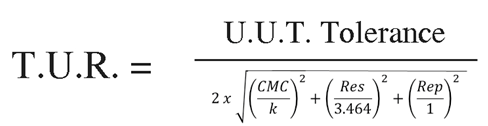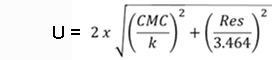How to Calculate Resolution of a Force-Measuring Device

Per Section 4.14 of JCGM 200:2012, Resolution is the “smallest change in a quantity being measured that causes a perceptible change in the corresponding indication.”   Over the years, this simple definition has become a topic of confusion amongst the metrology community.  We are hoping to simplify how to calculate the resolution of the Unit Under Test (UUT)  so this can be a simple guidance document.  However, we are first going to briefly discuss why calculating the resolution of the Unit Under Test properly is essential.

Morehouse reports the resolution of the Unit Under Test on our calibration certificates as well as uses the resolution of  in the divisor for calculating TUR and reporting Expanded Uncertainty.  TUR is defined in ANSI/NCSLI Z540.3-2006 and ILAC G8, as “The ratio of the span of the tolerance of a measurement quantity subject to calibration, to twice the 95 % expanded uncertainty of the measurement process used for calibration”.  This calculation of TUR is crucial as it is the basis for making statements of conformity required by ISO/IEC 17025:2017section 7.8.6.1 & 2.Figure 1 The typical minimum contributors to calculate TUR at the time of calibration

We typically deal with the resolution of the UUT in the denominator, as shown in figure 1 above.  ANSI/NCSL Z540.3 Handbook states, “For the denominator, the 95 % expanded uncertainty of the measurement process used for calibration per the calibration procedure is to be used to calculate TUR.  The value of this uncertainty, the estimate should reflect the results that are reasonably expected from the use of the approved procedure to calibrate the particular type of M&TE.  Therefore, the estimate includes all components of the error that have an influence on the measurement results of the calibration which would also include the influences of the item being calibrated, with the exception of the bias of the M&TE.  The calibration process error, therefore, includes temporary and non-correctable influences incurred during the calibration such as repeatability, resolution, error in the measurement source, operator error, error in correction factors, environmental influences, etc.”

ILAC P-14 addresses resolution as part of the Expanded Uncertainty in section 6.4 that states, “Contributions to the uncertainty stated on the calibration certificate shall include relevant short-term contributions during calibration and contributions that can reasonably be attributed to the customer’s device. Where applicable the uncertainty shall cover the same contributions to the uncertainty that were included in the evaluation of the CMC uncertainty component, except that uncertainty component evaluated for the best existing device shall be replaced with those of the customer’s device.”

Now that we’ve hopefully made a case for why we need to know the resolution of the UUT, we can discuss various methods for calculating the resolution.  Here at Morehouse, we often get asked, how do you calculate resolution?  The answer is we take the force applied value and divide it by the output of the UUT at the corresponding point, and multiply that number by the readability.  Let’s look at one example.  In Example 1, we have a 10,000 lbf load cell with an output of 4.11235 mV/V and the force-measuring device is read at the fifth decimal (0.00001), and the display counts by 1

 Example 1: Device Reads in mV/V & Counts by 1 Force Applied Measured Output of UUT Readability of UUT Count by 10,000 4.11235 0.00001 1 Resolution = ((10,000 / 4.11235) *0.00001)*1 = 0.02432

In our next example, we have a 10,000 lbf load cell with an output of 4.11235 mV/V and the force-measuring device is read at the fifth decimal  0.00001, and the display counts by 5

 Example 2: Device Reads in mV/V & Counts by 5 Force Applied Measured Output of UUT Readability of UUT Count by 10,000 4.11235 0.00001 5 Resolution = ((10,000 / 4.11235) *0.00001)*5 = 0.12158

In example 3,  we have multiple force points or numerous readings at the same force point; we would take the average of the force points and apply the same formula.

 Example 3: 2  Measured Values Device Reads in mV/V and Counts by 1 Force Applied Measured Output of UUT Measured Output of UUT Run 1 Run2 10,000 4.11235 4.11325 Average Output Readability of UUT Count by 4.1128 0.00001 1 Resolution = ((10,000 / 4.11228) *0.00001)*1 = 0.02431

We use this formula for all scenarios, even when the indicator counts in force units, as shown in example 4.

 Example 4: Device Reads in lbf Force Applied Measured Output of UUT Readability of UUT Count by 10,000 10250.5 0.1 5 Resolution= ((10,000 / 10,250.5) *0.1)*5 = 0.488

If we have multiple force points, we should then take the average resolution is shown in example 5.

 Example 5: Multiple Points mV/V Force Applied Measured Output of UUT Readability of UUT Count by Resolution 2,000 0.82199 0.00001 1 0.02433 4,000 1.64316 0.00001 1 0.02434 6,000 2.46741 0.00001 1 0.02432 8,000 3.28632 0.00001 1 0.02434 10,000 4.11235 0.00001 1 0.02432 Average Resolution 0.02433Figure 2 minimum contributors for Expanded Uncertainty calculation at the time of calibration for 95 % confidence

Note: Resolution may either be divided by a rectangular distribution or by the square root of 12 as shown above. Dilip Shah has written a lot more on the topic which can be found @ http://asq.org/quality-progress/2016/09/measure-for-measure/resolution-resolve.html. Dilip and Henry teach force classes with uncertainty at Morehouse instrument company. Anyone wishing to learn more should visit https://www.mhforce.com/Training/TrainingCourses

Conclusion:

In the five examples provided above, not much changes in the formula, we either take a single point value of the instruments measured value at capacity, use the formula for the average deflection values, or take the average over all of the points in the measuring range.   We multiply this value by the readability and then multiply by how much the instrument counts by.  The resolution is then used in reporting the Expanded Uncertainty, which is shown in Figure 2, which complies with the requirements of ILAC P-14 Policy for Uncertainty in Calibration.

Everything we do, we believe in changing how people think about force and torque calibration. Morehouse believes in thinking differently about force and torque calibration and equipment. We challenge the "just calibrate it" mentality by educating our customers on what matters, what causes significant errors, and focus on reducing them. Morehouse makes our products simple to use and user-friendly. And we happen to make great force equipment and provide unparalleled calibration services.

Wanna do business with a company that focuses on what matters most? Email us at info@mhforce.com.

Written by Henry Zumbrun

www.mhforce.com